# The surface area

How much percent will the surface area of a 4x5x8 cm block increase if the length of the shortest edge is increased by 2 cm?

p =  28.2609 %

### Step-by-step explanation: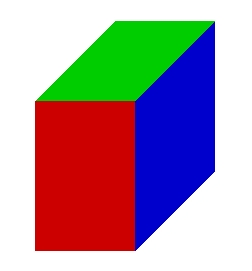Did you find an error or inaccuracy? Feel free to write us. Thank you!Tips to related online calculators

## Related math problems and questions:

• Cuboid - edgesThe cuboid has dimensions in ratio 4: 3: 5, the shortest edge is 12 cm long. Find: (A) the lengths of the remaining edges, (B) the surface of the cuboid, (C) the volume of the cuboid
• Carpenter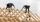From a wooden block carpenter cut off a small cuboid block with half the edge length. How many percent of wood did he cut off?
• Surface area 2Calculate how many % reduce the surface area of the cube is we reduced length of each edge by 10%.
• Cuboid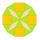The volume of the cuboid is 245 cm3. Each cuboid edge length can be expressed by an integer greater than 1 cm. What is the surface area of the cuboid?
• Cuboid edgesCalculate the volume and surface of a cuboid whose edge lengths are in the ratio 2: 3: 4 and the longest edge measures 10cm.
• Minimum surfaceFind the length, breadth, and height of the cuboid-shaped box with a minimum surface area, into which 50 cuboid-shaped blocks, each with length, breadth, and height equal to 4 cm, 3 cm, and 2 cm respectively, can be packed.
• Block or cuboidThe wall diagonals of the block have sizes of √29cm, √34cm, √13cm. Calculate the surface and volume of the block.
• PrismRight-angled prism, whose base is a right triangle with leg a = 3 cm and hypotenuse c = 13 cm, has the same volume as a cube with an edge length of 3 dm. a) Find the height of the prism b) Calculate the surface of the prism c) What percentage of the cube'
• Cube zoomHow many percents do we increase the volume and surface of the cube if we magnify its edge by 38 %?
• Magnification of the square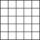If we increase the square side, increase the content of the 70 %. About what percentage was increased his sides?
• Volume increaseHow many percent will increase in the pool 50 m, width 15m if the level rises from 1m to 150cm?
• Solid cuboidA solid cuboid has a volume of 40 cm3. The cuboid has a total surface area of 100 cm squared. One edge of the cuboid has a length of 2 cm. Find the length of a diagonal of the cuboid. Give your answer correct to 3 sig. Fig.
• Cuboid - ratiosThe sizes of the edges of the cuboid are in the ratio 2: 3: 5. The smallest wall has an area of 54 cm2. Calculate the surface area and volume of this cuboid.
• Cuboid - simple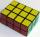Calculate the surface area and volume of a cuboid if a = 8 cm, b = 14 cm and c = 6 cm.
• Water blockA block with a 50 cm2 base is filled with water 5 cm under the edge. How many can sugar cubes with 2 cm edge be thrown into a container that overflow water?
• The body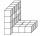The body on the figure consists of cubes with an edge length 10 cm. What surface has this body?
• Cuboid - ratioFind the volume of a block whose dimensions are in the ratio 2: 3: 4 and the surface is 117 dm2.